﻿﻿﻿ Pressure loss Bend 90° ﻿ ﻿ ﻿ ﻿

# Pressure loss in pipe bends

 Δ p = K · ρ/2 · v2 Δ p : Pressure loss of a bend 90º K : Resistance coefficient (Friction Factor) of a bend 90º ρ : Density v : Average Speed in the pipe Re : Reynolds number Re = ρ · v · d / η μ : Dyn. Viscosity d : Inner diameter of the bend

The Resistance coefficient in bends is a function of the Re-number, the roughness of the pipe wall and the bend geometry. A bend affects the flow pattern in front and behind the bend. The pressure drop of bends in series is lower or equal than the pressure drop calculated by adding the pressure loss of every single bend. TECCINESS assumes that the inner diameter of the pipe equals the inner diameter of the bend.

The Resistance coefficient for Re < 2320 (laminar flow) is calculated according to [Ghia 1977, page 648]. The graph plotted by Ghia has been approximated as follows:

 K / K,p = 0.026 · Dean ^0.661 +1 Kp = π/2 · r/d · 64/Re Resistance coefficientof a straight pipe with the length of the bend´s midline Dean = Re · (d/r)^0.5 Dean-number r Radius of the bend´s midline

The Resistance coefficient for Re > 2320 (transition or turbulent state) is calculated by a set of equations presented by [Krüger 1970, page 39 ff]. Krüger takes into consideration the bend radius and the wall roughness.

 ε /d 2320 < Re < 2 x 10^5 Re > 2 x 10^5 > 0.001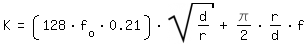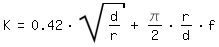< 0.001 Re > Re*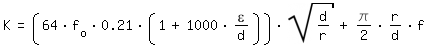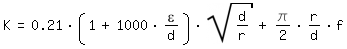Re < Re*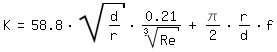0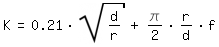f : Darcy Friction Factor of the straight pipe f,o : Darcy Friction Factor of a smooth straight pipe ε : roughness of the pipe wall Re* = (2,89/(1+1000 x ε /d))^12K = f(Re)

 Literature [Ghia 1977] K.N. Ghia, J.S. Sokhey, Laminar Incompressible Viscous Flow in Curved Ducts of Regular Cross-Sections, Transactions of the ASME Journal of Fluids Engineering, December 1977, page 640 ff [Kittredge 1957] C.P. Kittredge, D.S. Rowley, Resistance Coefficients for Laminar and Turbulent Flow Through One-Half-Inch Valves and Fittngs, Transactions of the American Society of Mechanical Engineering ASME, Volume 79 January 1957, Fig. 6 [Krüger 1970] H. Krüger, Berechnung strömungstechnischer Kennwerte von Durchströmteilen für Flüssigkeiten und Gase, Mitteilungen des Institut für Leichtbau und ökonomische Verwendug von Werkstoffen, IfL- Mitteilungen, Dresden 1970, Beilage [Miller 2008] D.S. Miller, Internal Flow Systems, 2nd Edition 2008, Miller Innovations Bredford UK, page 223 f [Ward Smith 1980] A.J. Ward-Smith, The fluid dynamics of flow in pipes and ducts, Clarendon Press, Oxford 1980, pages 248-268 [White 1929] C.M. White, Streamline flow through curvd pipes. Proc. Royal Soc., London, 123 (1929), page 645, cited by [Krüger 1970, page 39]

﻿